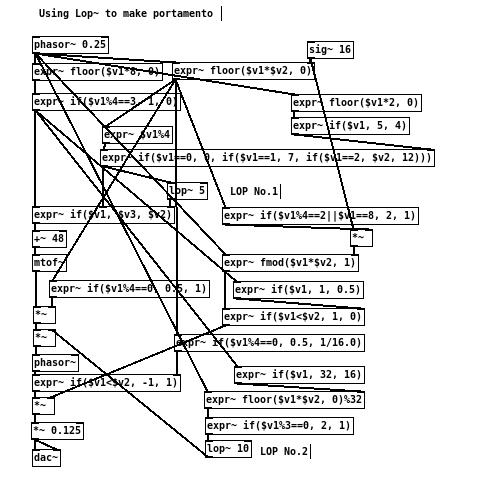```                  ______
/      |       ________
/       |____  /        \
/        |    \/   _      \
\       ___       /_\      \
\      \_/          .      \
\          /\_____/       /
\________/      |       /
|______/

```

# [THE_EXPR_METHOD] 008 Smooth lop~

20160317

By default, functions such as floor() or if() would produce a “hard” stepped signal. That is to say, signal changes abruptly from one level to the next, governed by predefined functions and timing. Whilst this behaviour is absolutely expected and correct, it may not results to the most “musical” effect at times. In other words, being able to move seamlessly from one value to another can contributes to a more “natural” quality to the composition. Musically, such an effect can be seen as “portamento”, where by the pitch slides from note to note, rather than stepped.

A simple one-pole low pass filter can be used in this case to “smooth” out incoming signal, making it much less abrupt. consider the following:

```[phasor~ 0.5] -> [expr~ (floor(\$v1*4, 0)+1)*200] -> [osc~] -> [dac~]
```

and

```[phasor~ 0.5] -> [expr~ (floor(\$v1*4, 0)+1)*200] -> [lop~ 10] -> [osc~] -> [dac~]
```

The value 10 given as the argument to [lop~] specifies the filter’s cut-off frequency, and in this case, it translates to the time taken to smooth out the incoming signal. For example, 1Hz would equates to 1 second in time, whereas 10Hz would only take 100 millisecond.

It is also worth noting that if the frequency given to [lop~] is lower/slower than the rate of the main timing, the resulting signal may never fully reach its target value. In this case, it would simple wobble along between different values.

Another use case for [lop~] would be a quick-and-dirty envelop set-up, such as:

```[phasor~ 1] -> [expr~ if(\$v1<0.5, 1, 0)] -> [lop~ 5]
```

Ordinarily, such type of “gate” switching would produce clicks at the edges of the on state. However, by passing the signal through a low pass filter, the hard edges thus are rounded off, making the transitions much less harsh.

The following example shows an simple riff using 2 [lop~]:Get the source here

Exercise:

• Are there any other use case for [lop~]
• What are the drawback of the [lop~] object in Pd used in this context?
• How about using other types of filters?